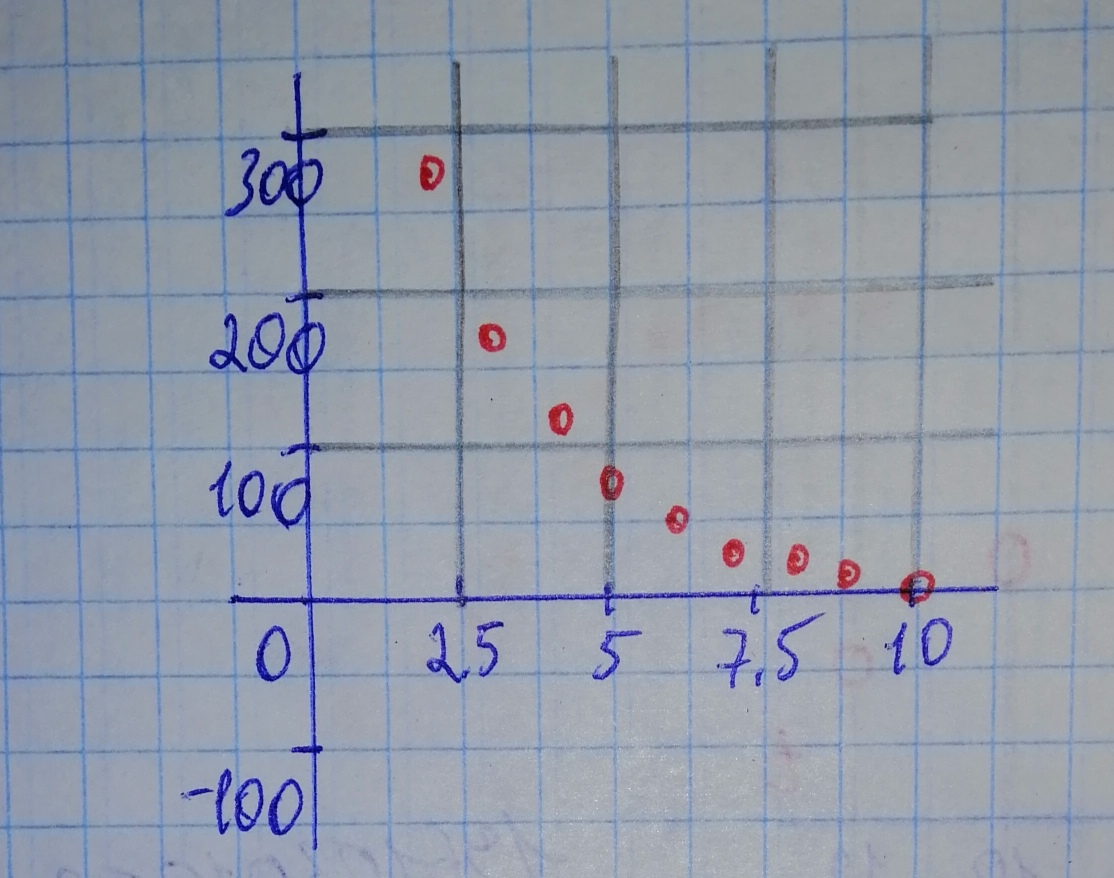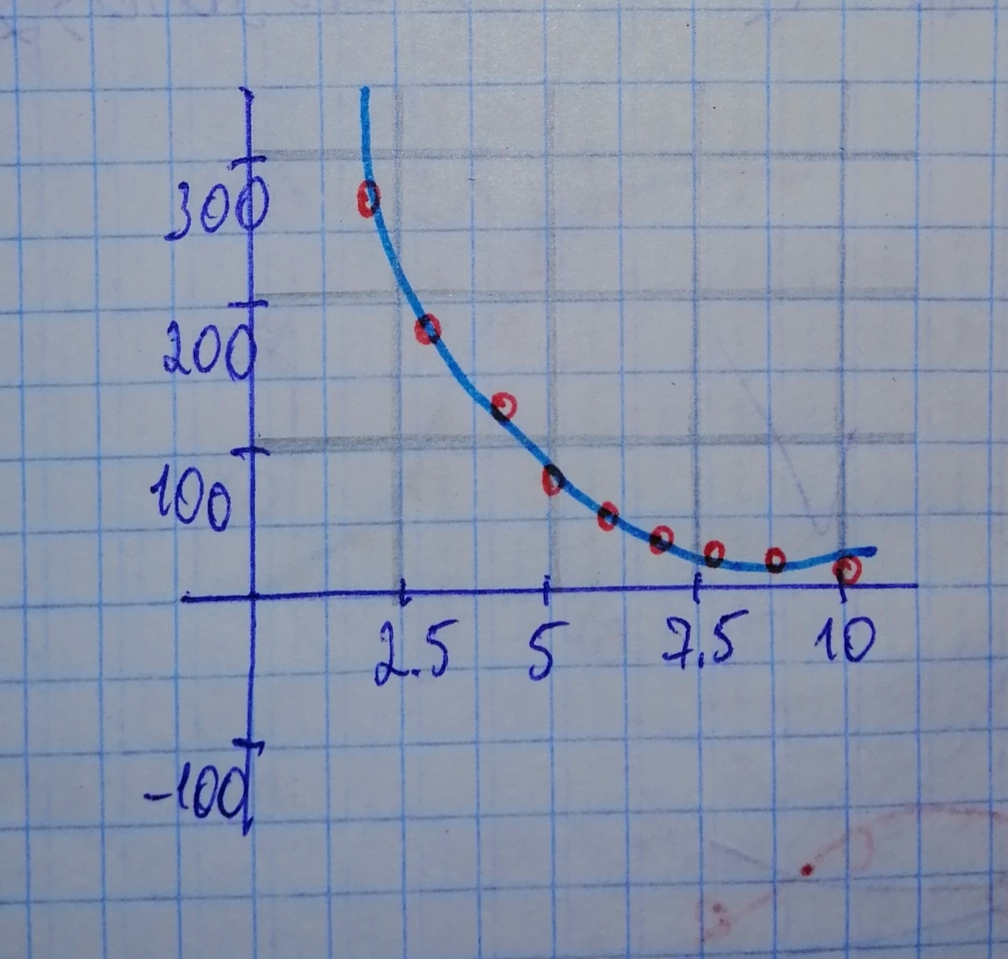ddaeeric

2021-02-18

For the following exercises, use a graphing utility to create a scatter diagram of the data given in the table. Observe the shape of the scatter diagram to determine whether the data is best described by an exponential, logarithmic, or logistic model. Then use the appropriate regression feature to find an equation that models the data. When necessary, round values to five decimal places.
$\begin{array}{|ccccccccccc|}\hline x& 1& 2& 3& 4& 5& 6& 7& 8& 9& 10\\ f\left(x\right)& 409.4& 260.7& 170.4& 110.6& 74& 44.7& 32.4& 19.5& 12.7& 8.1\\ \hline\end{array}$au4gsf

Step 1
Remember that regression analysis is the process of looking for a best fit of model for a set of data. This can be done on a graphing utility as follows:
1. Press [STAT], the input corresponging x-values of data in L1, and y-values of data in L2.
2. Use [STATPLOT] to observe a scatterplot of the data.
3. Press [STAT], then [CALC] then [ExpReg]/[LnReg]/[Logistic].
This will show you a function in either the form of an exponential, a logarithmic or a logistic model.
4. Graph this equation on the same window as the scatterplot to see if it fits the data.
Step 2
1. Press [STAT], the input corresponging x-values of data in L1, and y-values of data in L2.
2. Use [STATPLOT] to observe a scatterplot of the data.Step 3
Based on the plots of the points, it can be exponential or logarithmic.
However, upon checking both regression analysis, the one with the closest value of ${r}^{2}$ to 1 is exponential, hence, its formula is $y=628.67663{\left(0.64841\right)}^{x}.$ The graph of which is below:Do you have a similar question?